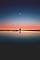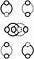# How a Simple Circle Runs the Whole Universe

Complementarity is the basis for identity because duplicity is the basis for a unit. Proving we already have a ‘theory of everything’ at our disposal.Complementarity is the basis for identity. (Photo by Jordan Steranka) The Theory of Everything.

Complementarity is the basis for identity because duplicity is the basis for a unit because a simple circle is conserved (meaning you cannot have ‘yin’ without ‘yang’ because you cannot have a circumference (zero) without a diameter (one)). This means a simple circle runs the whole universe. Explaining rotation, revolution, and radiation (electricity, magnetism, and gravity) (everything in Nature).

This, also, explains the core relationship between hydrogen and oxygen. Hydrogen, when burned, produces water, meaning, you cannot atomize these, or separate, elements, at all. A simple circle joins and separates any X and-or Y. This changes how we think about chemistry.

Only a circle can circle. (A circle can only circle.) Meaning ‘circle’ is the correct (underlying) ‘name’ (symbol) for any (and every) entity, and-or process (noun and-or verb). The correct name (articulation) for a ‘unit.’ Any size or scale.

X and Y is X and X. Meaning, X and X’ breaks the law of identity. This is why, and how, technology has exploded from, into, and because of, the zero and the one (technically, circumference and diameter).

A universe is more correctly called a circle. Which is the technical definition for the constructions, and structures, humans use to define, elaborate, and, also, isolate, the number ‘one’ (number systems and alphabet systems) (symbolic systems in general).

This means a basic unit, including a human unit, is bound together as a zero and a one. In technological terms, if zero, then one, meaning, if one, then zero. This explains life and death in all systems. Mind as a completely relative structure called ‘square one.’X and Y. X and X. X and X’. (Square one).

Therefore, from a technological point of view, meaning, from a technical point of view, universal relativity is universal circularity, because the constant is a variable (and vice versa), meaning, from a human’s point of view, there are only two constants in nature: a zero, and a one.

This means we can only have two states in nature. A zero. Or a one. Meaning, the white hole you see up in the sky (sun, and-or, moon) (ancient yang, and-or, yin) is a zero, and-or, a one. Explaining why energy (a wave) occurs in circles. If the constant is a zero, no energy, no mass (pure space) (correct definition for the most basic unit of space). If the constant is a one, energy is mass (and vice versa).

Producing (explaining, proving) (controlled by) a ‘universal system architecture.’

This is the technical explanation for a black hole, a universe, a star, which is, more correctly defined, and structured, as, a, circle (noun, and verb) (a circle of circles is still a circle) (eliminating the need for spheres, and radii) (areas and volumes) (black holes and universes) (solar systems altogether).

Therefore, zero and one control reality, including a human’s perception of reality, and a human’s definition of reality, and a human’s participation in reality. Again, if zero, then one. Meaning, if one, then zero.

Mind and matter (abstract and concrete) (symbolic systems in general) articulate, and, thus, conserve, and are controlled by, an uber-simple circle. Explaining both magnetism and electricity (why we attract, and, then, down the road, definitely, repel) (why everything is 50–50) (no matter what we do).

Meaning, if you think about it carefully, pi is the only observer.

Proving, we already have (and know all about) the ‘Theory of Everything’ at our disposal. Yin and yang (ancient) is zero and one (modern) meaning the oppositional dynamic forms the basis for reality. So, why are we still ‘searching?’

Conservation of the circle is the core dynamic in nature.

If you consider yourself a ‘thought leader…

## The Circular Theory

### By The Circular Theory

Conservation of the circle is the core, and, therefore, the only dynamic in Nature. Meaning, pi, in mathematics, is the technical name for, what a human labels, 'mind.'  Take a look

Written by

Written by

## Ilexa Yardley

#### Author, The Circular Theory